13. The inner approximation.

We rescale the problem by puttingin our original differential equationIfwe getThe equation then transforms toConsider the coefficientsThe problem in the original equation is that the coefficient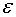of the highest derivative y” is small compared to the others. To avoid this problem we thus choose the main coefficientto be of the same order as one of the other coefficients and that the other two coefficients are comparably small. We demonstrate the procedure below (remember thatis small):

 Case 1):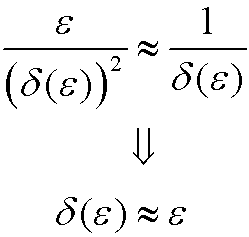Case 2):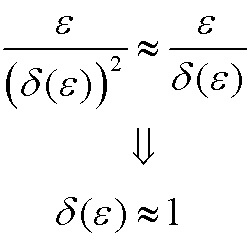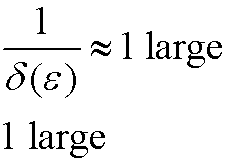Case 3):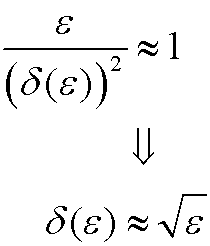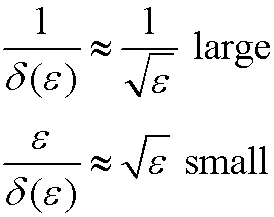Ve see that we only have one possibility and that is Case 1, where the main coefficient is relatively larger than the remaining coefficients. We therefore chooseOur transformed equation then becomes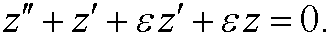If we now putwe get the equationwith the solution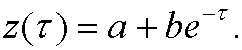The boundary condition z(0)=y(0)=0 then yields thatOur inner approximation is thusThe remaining problem is thus to determine the constant a and match the inner and outer approximations.